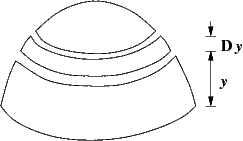Consider the volume of the region shown below, which shows a hemisphere of radius 3 mm and a slice of the hemisphere with width $Dy = \Delta y$.Write a Riemann sum for the volume, using the slice shown:
Riemann sum $= \Sigma$

Now write an integral that gives this volume
$\int_a^b\,$
where $a =$ and $b =$

Finally, calculate the exact volume of the region, using your integral
volume =
(include units)

You can earn partial credit on this problem.